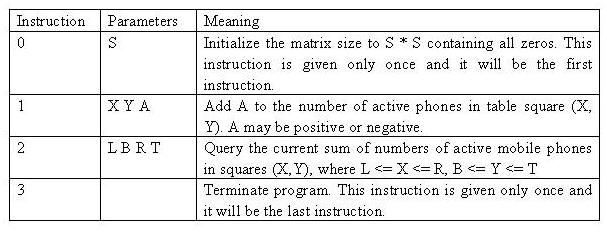Online JudgeProblem SetAuthorsOnline ContestsUser
Web Board
F.A.Qs
Statistical Charts
Problems
Submit Problem
Online Status
Prob.ID:
Register
Authors ranklist
Current Contest
Past Contests
Scheduled Contests
Award Contest
Register
Language:
Mobile phones
 Time Limit: 5000MS Memory Limit: 65536K Total Submissions: 27213 Accepted: 12362

Description

Suppose that the fourth generation mobile phone base stations in the Tampere area operate as follows. The area is divided into squares. The squares form an S * S matrix with the rows and columns numbered from 0 to S-1. Each square contains a base station. The number of active mobile phones inside a square can change because a phone is moved from a square to another or a phone is switched on or off. At times, each base station reports the change in the number of active phones to the main base station along with the row and the column of the matrix.

Write a program, which receives these reports and answers queries about the current total number of active mobile phones in any rectangle-shaped area.

Input

The input is read from standard input as integers and the answers to the queries are written to standard output as integers. The input is encoded as follows. Each input comes on a separate line, and consists of one instruction integer and a number of parameter integers according to the following table.The values will always be in range, so there is no need to check them. In particular, if A is negative, it can be assumed that it will not reduce the square value below zero. The indexing starts at 0, e.g. for a table of size 4 * 4, we have 0 <= X <= 3 and 0 <= Y <= 3.

Table size: 1 * 1 <= S * S <= 1024 * 1024
Cell value V at any time: 0 <= V <= 32767
Update amount: -32768 <= A <= 32767
No of instructions in input: 3 <= U <= 60002
Maximum number of phones in the whole table: M= 2^30

Output

Your program should not answer anything to lines with an instruction other than 2. If the instruction is 2, then your program is expected to answer the query by writing the answer as a single line containing a single integer to standard output.

Sample Input

```0 4
1 1 2 3
2 0 0 2 2
1 1 1 2
1 1 2 -1
2 1 1 2 3
3
```

Sample Output

```3
4```

Source

[Submit]   [Go Back]   [Status]   [Discuss]Home PageGo BackTo top